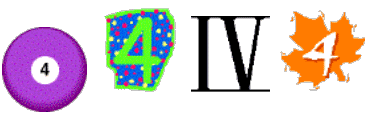#### You may also like### Cubes

How many faces can you see when you arrange these three cubes in different ways?### Pebbles

Place four pebbles on the sand in the form of a square. Keep adding as few pebbles as necessary to double the area. How many extra pebbles are added each time?### Bracelets

Investigate the different shaped bracelets you could make from 18 different spherical beads. How do they compare if you use 24 beads?

# Four Goodness Sake

##### Age 7 to 11 Challenge Level:Write down the number $4$, four times.
Put operation symbols between them so that you have a calculation.
So you might think of writing $4 \times 4 \times 4 - 4 = 60$

#### BUT use operations so that the answer is $12$

Now, can you redo this so that you get $15$, $16$ and $17$ for your answers?

Need more of a challenge? Try getting answers all the way from $0$ through to $10$.